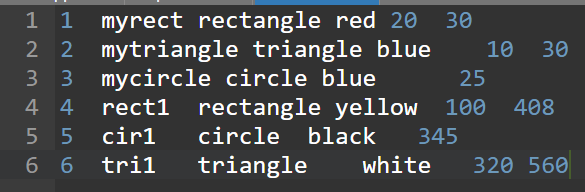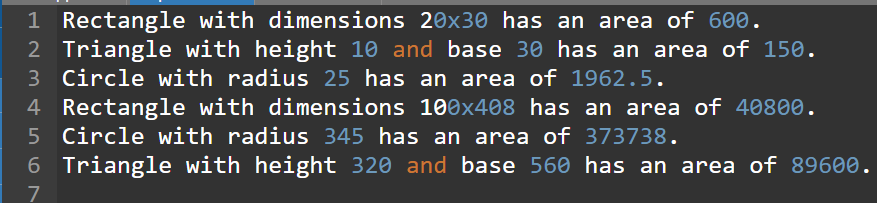# It’s C++, I have to create a program that can make a text file, open it, and read the information.

## Question

It’s C++, I have to create a program that can make a text file, open it, and read the information. That text file should have information such as if an object is a circle, rectangle, or triangle, with the length of the sides. The idea of the program is to read that text file, check which type of shape is and the length, find the area or radius, and then write that information into another text file. I’m going to send the code I have right now, but I don’t know how to modify the program so it can create the txt file, read the values, check which object it is, and write that in a new txt file.

#include <iostream>
#include<fstream>
#include<string>
using namespace std;
class Shape{
protected: double x, y;
public: void set_dim(double i, double j=0){
x = i;
y = j;
}
virtual void show_area(void){
cout << "No area computation defined ";
cout << "for this class.\n";
}
} ;
class triangle : public Shape{
public: void show_area(void){
cout << "Triangle with height ";
cout << x << " and base " << y;
cout << " has an area of ";
cout << x * 0.5 * y << ".\n";
}
};
class square : public Shape{
public: void show_area(void){
cout << "Square with dimensions ";
cout << x << "x" << y;
cout << " has an area of "; cout << x * y << ".\n";
}
};
class circle : public Shape{
public: void show_area(void){
cout << "Circle with radius ";
cout << x;
cout << " has an area of ";
cout << 3.14 * x * x;
}
} ;
int main(void){
Shape *p;
triangle t;
square s;
circle c;
p = &t;
p->set_dim(10.0, 5.0);
p->show_area();
p = &s;
p->set_dim(10.0, 5.0);
p->show_area();
p = &c;
p->set_dim(9.0);
p->show_area();
return 0;
}


1. Write a class of Shape that has two member variables, perimeter and area, and pure virtual functions to calculate the perimeter and area of the shape. It also contains two additional member variables:

a. m_name: a string with no more than 10 characters for the name of the object

b. m_ID: an integer index of the object.

2. Write a class of Color with a member variable that can be red, yellow, blue, black, and white.

3. Write three classes for rectangle, triangle, and circle objects, derived from the class of Shape and Color for objects with the shape of the triangle, rectangle, or circle, and any of the colors defined in the class of Color.

4. Write necessary constructor/destructor functions, accessor/mutator functions for each class.

a. print: print the pertinent information for a given shape and color

b. calculate: calculate the area and perimeter for the current shape

5. Your program should read the object info from an input file called input-3-3.txt, which lists the index, object name, color, length of each side (radius if it is a circle). similar as follows:

1  myrect  rectangle  red  20  30

2  mytriangle  triangle  blue  10  30  35

3  mycircle  circle  blue  25

4  rect2  rectangle   blue  30  40

6. Your program needs to calculate the perimeter and area of each object, and set the object’s color to be black if its area is larger than the overall average of all objects and white otherwise. Your program should output the following information to another file called output-3-3.txt. Please name your main file as prog-3-3.cpp.

a. The overall average area of all objects

b. The index, name, perimeter, area, color

## Summary

Basically, a base class shape is defined with a virtual function show_area, and another function set_dim, which are redefined by all the other derived classes of shape. They are rectangle, circle, and triangle. Each class has its own definition for the area. The major thing done, is to read the input for each shape from an input file named ‘data.txt’ and write the details of the area to an output file.

## Explanation

We have defined the class Shape with two protected data members with the names x and y. We have defined the function set_dim to set the dimensions of the shape. The function set_area is defined to find the area of each shape and it is made virtual. The derived class rectangle, circle, and triangle are defined which have a copy of the protected variables of the Shape class.

All these classes have their specific definition for the show_area with the required formula to calculate the area. A file named ‘data.txt’ is opened and each record is read to detect the shape, and according to that class object calls the set_dim function and specifies the dimensions, and the show_area function is called to which an ofstream object is passed, to which the area output is written. The output file is ‘output.txt’.

## Code

#include <iostream>
#include<fstream>
#include<string>
using namespace std;
/* base class with virtual function */
class Shape {
protected: double x, y;
public: void set_dim(double i, double j=0){
x = i;
y = j;
}
virtual void show_area(ofstream& out){
out << "No area computation defined ";
out << "for this class.\n";
}
} ;
/* first derived class for shape triangle */
class triangle : public Shape{
public: void show_area(ofstream& out){
out << "Triangle with height ";
out << x << " and base " << y;
out << " has an area of ";
out << x * 0.5 * y << ".\n";
}
};
/* second derived class for shape rectangle */
class rectangle : public Shape{
public: void show_area(ofstream& out){
out << "Rectangle with dimensions ";
out << x << "x" << y;
out << " has an area of "; out << x * y << ".\n";
}
};
/* thrid derived class for shape circle */
class circle : public Shape{
public: void show_area(ofstream& out){
out << "Circle with radius ";
out << x;
out << " has an area of ";
out << 3.14 * x * x<<".\n";
}
} ;

int main(void){
Shape *p;
triangle t;
rectangle r;
circle c;
/* to read the input file */
ifstream in; in.open("data.txt");
/* to write the output file */
ofstream out; out.open("output.txt");
int x;
while(in>>x){
string object;  in>>object;
string shape; in>>shape;
string color; in>>color;
/* for rectangle */
if(shape=="rectangle"){
int x,y; in>>x>>y;
p=&r; p->set_dim(x,y); p->show_area(out);
}
/* for circle */
else if(shape=="circle"){
int r; in>>r;
p=&c; p->set_dim(r); p->show_area(out);
}
/* for triangle */
else if(shape=="triangle"){
int b,h; in>>b>>h;
p=&t; p->set_dim(b,h); p->show_area(out);
}
}
}


## Output